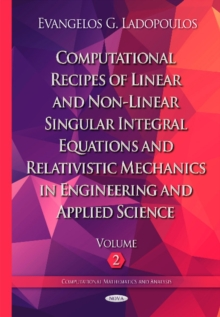Supporting your high street Find out how »
• My Account# Computational Recipes of Linear & Non-Linear Singular Integral Equations & Relativistic Mechanics in Engineering & Applied Science : Volume II Hardback

#### Description

This book deals with the computational recipes of the finite-part singular integral equations, the multidimensional singular integral equations and the non-linear singular integral equations, which are widely used in many fields of engineering mechanics and mathematical physics with an applied character, like elasticity, plasticity, thermoelastoplasticity, viscoelasticity, viscoplasticity, fracture mechanics, structural analysis, elastodynamics, fluid mechanics, potential flows, hydraulics and aerodynamics.

Such types of linear and non-linear singular integral equations form the latest technology of very important problems of solid and fluid mechanics, which should be given special attention by the reader.

The Singular Integral Operators Method (S.I.O.M.) is introduced and investigated for the numerical evaluation of the multidimensional singular integral equations.

This approximation method in many cases offers important advantages over "domain" type solutions, like finite elements and finite difference, as well as analytical methods such as complex variable methods.

Additionally, a special field of applied mechanics is introduced, named as Relativistic Mechanics, which is a combination of the classical theory of elasticity and general relativity.

Relativistic Mechanics has two main branches: Relativistic Elasticity and Relativistic Thermo-Elasticity and according to the above theory, the relative stress tensor for moving structures has been formulated and a formula has been given between the relative stress tensor and the absolute stress tensor of the stationary frame.

This leads to the Universal Equation of Elasticity and the Universal Equation of Thermo-Elasticity.

#### Information

• Format: Hardback
• Pages: 265 pages
• Publisher: Nova Science Publishers Inc
• Publication Date:
• Category: Calculus & mathematical analysis
• ISBN: 9781634824514

£252.99

£201.75£270.99

£215.95